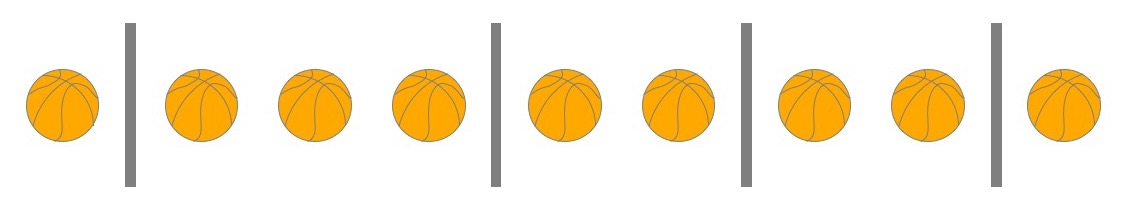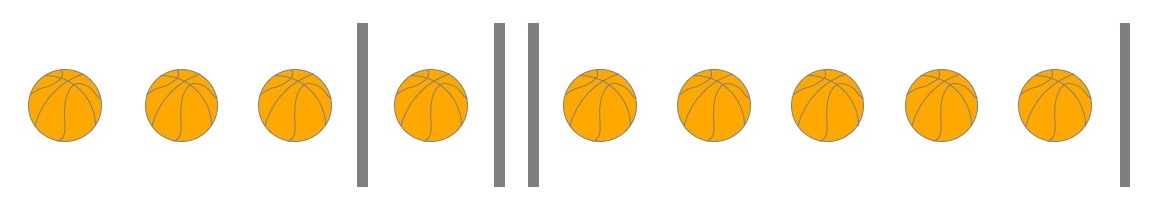# Moving Day - Solution

The first thing we notice is that the choices for the basketballs, volleyballs, baseballs, and golf balls are all independent: how Leslie assigns one type of ball to the different trucks doesn’t restrict how she might assign a different type of ball to the different trucks. So we can start with just the basketballs and hope that we can re-use our technique on the other kinds of balls.

Examining just the basketballs, we next might notice that all the balls are the same: they’re indistinguishable. The trucks, on the other hand, are numbered and therefore are distinguishable. That means assigning all 9 basketballs to Truck 1 is different from assigning all 9 basketballs to Truck 5, but if two trucks both contain basketballs, swapping a basketball from one truck with a basketball from the other truck doesn’t change anything.

How does this help us? It means that Leslie can line up all the basketballs in a row, put down “dividers” at some points in the lineup, and give the resulting groups basketballs in between the dividers to the corresponding trucks. What’s clever about this setup is that Leslie’s choice of where the dividers are placed corresponds exactly to an assignment of basketballs to trucks. In particular, basketballs are still indistinguishable (swapping two of them in the lineup doesn’t change anything about the placement of the dividers) and trucks are still distinguishable (to change which trucks get which numbers of basketballs, you have to change where the dividers are placed).

Okay, so now we’ve lined up all the basketballs and we’re choosing where to put the dividers. How many dividers do we need? After a bit of thinking, we decide that 4 is plenty: there are 5 trucks, so there are 4 “gaps between the trucks.” The groups of basketballs at either end of the lineup won’t have a divider on the other side of them, but that’s fine – we’ll just know that when we get to the end of the line, we’re done with the group. Here’s what this looks like: to assign 1 basketball to Truck 1, 3 to Truck 2, 2 each to Trucks 3 and 4, and 1 to Truck 5, Leslie can place dividers like so:“I get it,” you might say at this point. “There are 9 basketballs, so there are 8 ‘gaps between the basketballs’ in which the dividers might go, and we want to pick 4 of these gaps to contain dividers, so there are ${8 \choose 4}$ ways to do this.”

Hold on, though! You’re close, but there’s a catch: some trucks might not be assigned any basketballs at all. This corresponds to having multiple dividers in the same “gap between basketballs” or having one or more dividers at the end of a row. For instance, to assign 3 basketballs to Truck 1, 1 to Truck 2, 5 to Truck 4, and none to Trucks 3 or 5, Leslie can place dividers like so:So we’re not quite done. Here’s how we fix it: we put down 9 + 4 “placeholders” that could each hold either a basketball or a divider. Then we choose 4 of those placeholders to contain dividers, and the rest of the placeholders will contain basketballs. Now we can have two dividers in adjacent placeholders, meaning a truck with zero basketballs. Think this over some more and make sure you understand why this fixes the problem in our first attempted solution.

So there are ${9+4 \choose 4}$ ways to assign the basketballs to the 5 trucks. Does this approach work for the other types of balls also? Luckily, yes! Following the same reasoning, we can determine that there are ${61+4 \choose 4}$ ways to assign the volleyballs, ${88+4 \choose 4}$ ways to assign the baseballs, and ${323+4 \choose 4}$ ways to assign the golf balls. Since all of these choices are independent, we multiply them to get a final answer of

$${13 \choose 4}{65 \choose 4}{92 \choose 4}{327 \choose 4}$$

ways to assign all the balls to the five trucks. That’s a pretty big number!

(By the way, this kind of problem comes up fairly often when counting things. You might see it called “balls in boxes” or “stars and bars” in other contexts.)

As a twist on the problem, suppose the driver of Truck 3 really doesn’t like golf and doesn’t want to transport any of the golf balls. Now how many ways are there for Leslie to assign the balls to the trucks? Can you find the general formula for the number of ways to assign balls to trucks if there are $n$ indistinguishable balls and $k$ distinguishable trucks?